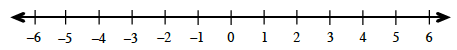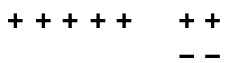### Home > MC2 > Chapter 2 > Lesson 2.1.2 > Problem2-26

2-26.

Find the missing value in each number sentence.

1. $4 + (-3) + 3 - l =$____

Draw a number line. Start at $0$.1. $-2 - (-4) + 4 =$____1. $5 -$ ____ $= 7$

This represents $5$:What do you need to take away so that $7$ remains?

Use the eTool below to model each number sentence.
Click the link at right for the full eTool version: MC2 2-26 HW eTool